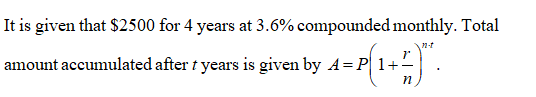# Use the compound interest formula to compute the total amount accumulated and the interest earned.\$2500 for 4 years at 3.6​% compounded monthlyThe total amount accumulated after 4yrs is \$_____Round to the nearest cent as needed

Question
1 views

Use the compound interest formula to compute the total amount accumulated and the interest earned.

\$2500 for 4 years at 3.6​% compounded monthly

The total amount accumulated after 4yrs is \$_____

Round to the nearest cent as needed

check_circle

Step 1Step 2

Here, A is the total amount, P is the principal, r is the interest rate, n is the number of compounding’s .That is,

Step 3

Obtain the total amount accumu...

### Want to see the full answer?

See Solution

#### Want to see this answer and more?

Solutions are written by subject experts who are available 24/7. Questions are typically answered within 1 hour.*

See Solution
*Response times may vary by subject and question.
Tagged in

### Math